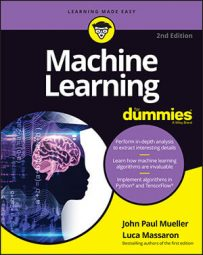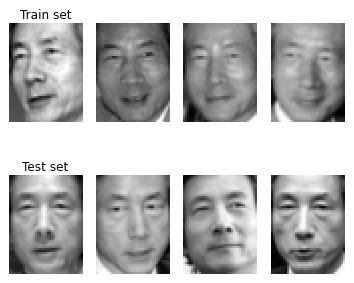##### Machine Learning For DummiesYou can apply a machine learning algorithm to a complex set of images, called the Labeled Faces in the Wild dataset that contains images of famous people collected over the Internet. You must download the dataset from the Internet, using the Scikit-learn package in Python. The package mainly contains photos of well-known politicians.

`import warnings`

`warnings.filterwarnings("ignore")`

`from sklearn.datasets import fetch_lfw_people`

`lfw_people = fetch_lfw_people(min_faces_per_person=60,`

`resize=0.4)`

`X = lfw_people.data`

`y = lfw_people.target`

`target_names = [lfw_people.target_names[a] for a in y]`

`n_samples, h, w = lfw_people.images.shape`

`from collections import Counter`

`for name, count in Counter(target_names).items():`

`print ("%20s %i" % (name, count))`

` `

` `

`Ariel Sharon 77`

`Junichiro Koizumi 60`

`Colin Powell 236`

`Gerhard Schroeder 109`

`Tony Blair 144`

`Hugo Chavez 71`

`George W Bush 530`

`Donald Rumsfeld 121`

As an example of dataset variety, after dividing the examples into training and test sets, you can display a sample of pictures from both sets depicting Jun’Ichiro Koizumi, Prime Minister of Japan from 2001 to 2006.

`from sklearn.cross_validation import`

`StratifiedShuffleSplit`

`train, test = list(StratifiedShuffleSplit(target_names,`

`n_iter=1, test_size=0.1, random_state=101))`

` `

`plt.subplot(1, 4, 1)`

`plt.axis('off')`

`for k,m in enumerate(X[train][y[train]==6][:4]):`

`plt.subplot(1, 4, 1+k)`

`if k==0:`

`plt.title('Train set')`

`plt.axis('off')`

`plt.imshow(m.reshape(50,37),`

`cmap=plt.cm.gray, interpolation='nearest')`

`plt.show()`

` `

`for k,m in enumerate(X[test][y[test]==6][:4]):`

`plt.subplot(1, 4, 1+k)`

`if k==0:`

`plt.title('Test set')`

`plt.axis('off')`

`plt.imshow(m.reshape(50,37),`

`cmap=plt.cm.gray, interpolation='nearest')`

`plt.show()`Examples from the training and test sets do differ in pose and expression.

As you can see, the photos have quite a few variations, even among photos of the same person, which makes the task challenging: expression, pose, different light, and quality of the photo. For this reason, the example that follows applies the eigenfaces method, using different kinds of decompositions and reducing the initial large vector of pixel features (1850) to a simpler set of 150 features.

The example uses PCA, the variance decomposition technique; Non-Negative Matrix Factorization (NMF), a technique for decomposing images into only positive features; and FastIca, an algorithm for Independent Component Analysis, an analysis that extracts signals from noise and other separated signals (the algorithm is successful at handling problems like the cocktail party problem).

`from sklearn import decomposition`

`n_components = 50`

`pca = decomposition.RandomizedPCA(`

`n_components=n_components,`

`whiten=True).fit(X[train,:])`

`nmf = decomposition.NMF(n_components=n_components,`

`init='nndsvda',`

`tol=5e-3).fit(X[train,:])`

`fastica = decomposition.FastICA(n_components=n_components,`

`whiten=True).fit(X[train,:])`

`eigenfaces = pca.components_.reshape((n_components, h, w))`

`X_dec = np.column_stack((pca.transform(X[train,:]),`

`nmf.transform(X[train,:]),`

`fastica.transform(X[train,:])))`

`Xt_dec = np.column_stack((pca.transform(X[test,:]),`

`nmf.transform(X[test,:]),`

`fastica.transform(X[test,:])))`

`y_dec = y[train]`

`yt_dec = y[test]`

After extracting and concatenating the image decompositions into a new training and test set of data examples, the code applies a grid search for the best combinations of parameters for a classification support vector machine to perform a correct problem classification.

`from sklearn.grid_search import GridSearchCV`

`from sklearn.svm import SVC`

`param_grid = {'C': [0.1, 1.0, 10.0, 100.0, 1000.0],`

`'gamma': [0.0001, 0.001, 0.01, 0.1], }`

`clf = GridSearchCV(SVC(kernel='rbf'), param_grid)`

`clf = clf.fit(X_dec, y_dec)`

`print ("Best parameters: %s" % clf.best_params_)`

` `

`Best parameters: {'gamma': 0.01, 'C': 100.0}`

After finding the best parameters, the code checks for accuracy — the percentage of correct answers in the test set — and obtains an estimate of about 0.82 (the measure may change when you run the code on your computer).

`from sklearn.metrics import accuracy_score`

`solution = clf.predict(Xt_dec)`

`print("Achieved accuracy: %0.3f"`

`% accuracy_score(yt_dec, solution))`

` `

`Achieved accuracy: 0.815`

More interestingly, you can ask for a confusion matrix that shows the correct classes along the rows and the predictions in the columns. When a character in a row has counts in columns different from its row number, the code has mistakenly attributed one of the photos to someone else. In the case of the former Prime Minister of Japan, the example actually gets a perfect score (notice that the output shows a 6 in row 6, column 6, and zeroes in the remainder of the entries for that row).

`from sklearn.metrics import confusion_matrix`

`confusion = str(confusion_matrix(yt_dec, solution))`

`print (' '*26+ ' '.join(map(str,range(8))))`

`print (' '*26+ '-'*22)`

`for n, (label, row) in enumerate(`

`zip(lfw_people.target_names,`

`confusion.split('\n'))):`

`print ('%s %18s > %s' % (n, label, row))`

` `

`0 1 2 3 4 5 6 7`

`----------------------`

`0 Ariel Sharon > [[ 6 0 1 0 1 0 0 0]`

`1 Colin Powell > [ 0 22 0 2 0 0 0 0]`

`2 Donald Rumsfeld > [ 0 0 8 2 1 0 0 1]`

`3 George W Bush > [ 1 1 2 46 1 0 0 2]`

`4 Gerhard Schroeder > [ 0 0 2 1 6 1 0 1]`

`5 Hugo Chavez > [ 0 0 0 0 1 5 0 1]`

`6 Junichiro Koizumi > [ 0 0 0 0 0 0 6 0]`

`7 Tony Blair > [ 0 0 0 1 2 0 0 11]]`